# Tools That Can be Used in Control System Design

Designing control systems needs a tool to speed up the task. Here are the tools that can be used in control system design. Matlab control systems toolbox is in the top of the list then followed by Simulink control design. I also included here simpowersystems because of its usefulness in power electronics. Control system is not as easy as ohms law. In fact as an SMPS design engineer I consider this as top of the list in terms of complexity. In SMPS all the switching converters need to have a good control so that it can regulate as expected. I combined these tools to design a good controller and of course to decrease the lead time of manual computation.

## Top 6 Tools That Can be Used in Control System Design

### 1. Matlab Control System Toolbox

I put Matlab control system toolbox in the top list of the tools that can be used in control system design. May be because of its popularity in universities and field of research. Matlab is the number 1 option if you know the transfer function of the plant. I remember during my college days what we used was matlab also but the pure scripting method. On the newer version of Matlab there is the so called SISO tool incorporated with Matlab control system toobox. If you have a ready plant transfer function, you can export it to SISO tool to start optimizing a controller based on that plant. In the matlab workspace you can process the plant transfer function e.g. from z domain to s domain or vice versa. Below are the SISO tool windows. They will appear when you load the SISO tool.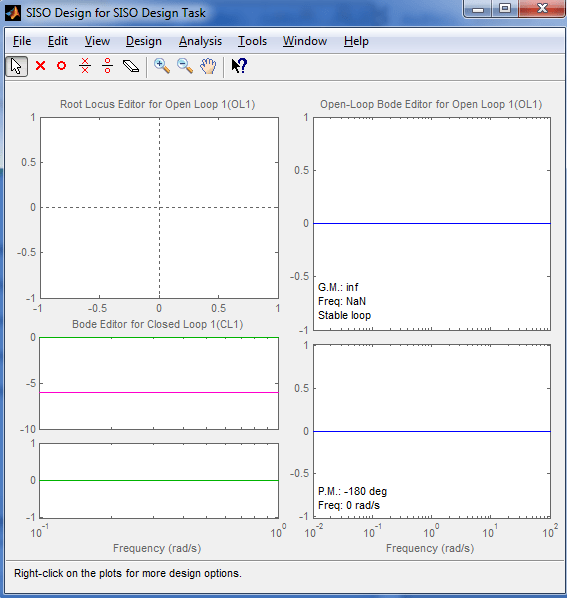Figure 1: SISO Design for SISO Design Task

`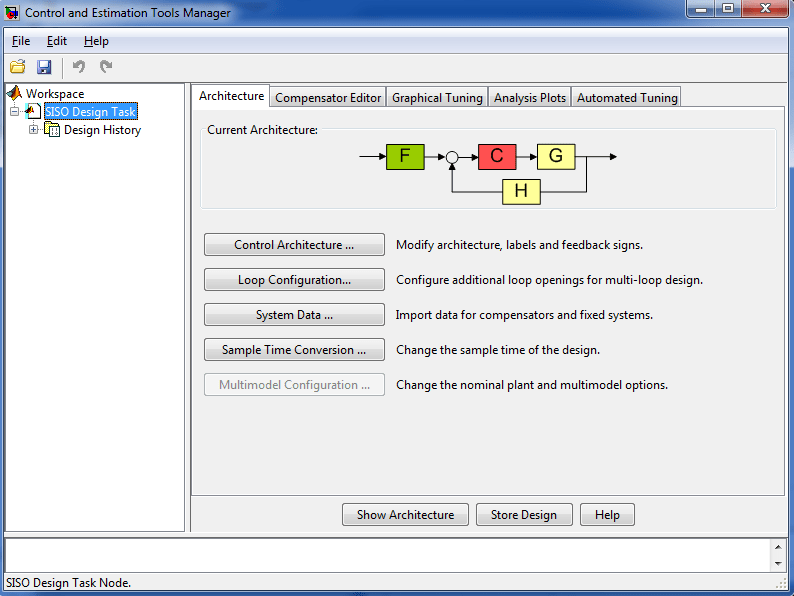`
Figure 2: Control and Estimation Tools Manager

#### SISO Design for SISO Design Task

The “SISO Design for SISO Design Task” is empty in the beginning. It will be filled when you import a transfer function from workspace.

#### Three Sections of SISO Design for SISO Design Task

A. Root Locus Editor for Open loop

The upper left area is the Root Locus Editor for open loop wherein you can tune a particular controller.

B. Bode Editor for Closed Loop

The bottom left windows are the close loop gain and phase response. You can see the response of the entire system here.

C. Open Loop Bode Editor for Open Loop

This window will display the open loop response

Control and Estimation Tools Manager

In this window you can choose the control architecture do you prefer. Once you click the “Control Architecture” button under “Architecture” tab.

For complete tutorials on how to use Matlab for control design click here.

Second on the list of the top tools that can be used in control system design is the Simulink control design. If using SISO tool is simple when the transfer function is known, by using SIMULINK is much simpler than that. Simulink is a model based environment within Matlab. When you buy a Matlab license only; this toolbox is not included. You need to buy also Simulink and of course Simulink Control Design toolbox. Basically this is a part of matlab that you can do drag and drop simulations (model based). Below is the Simulink Library browser. This is the window that pops up first when you open the Simulink environment.

``
Figure 3 : Simulink Library Browser

As you can see, the Library browser contains blocks. If you click on these blocks, there are further sub blocks inside that you can drag to the new model and use in your simulation. You can get these blocks also in the Libraries in the left.

Below is an example new model I made. I get all these blocks from the library. I used PID as controller to my plant which is represented by a transfer function. The purpose of the scope is to view the response of the system on a step input.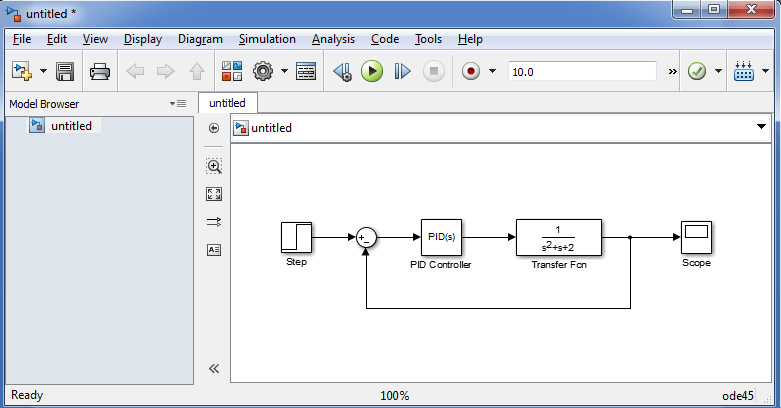If I will double click the PID block, below window will appear. In this window you can choose a controller whether you stick with the default PID or you will use PI or whatever. You can select either continuous or discrete time as well. The compensator formula is also shown at the right.Figure 5: PID Controller Attributes

If you are finish making selections, you can click the Tune button to start tuning. Below window will show.Figure 6: Simulink Auto Tuning Window

Unlike the SISO tool tuning which is using the root locus approach that is a bit tiresome, here the tuning is very easy. All you need to do is move the tuning bar in red box below. Once you already get the desired response it’s done.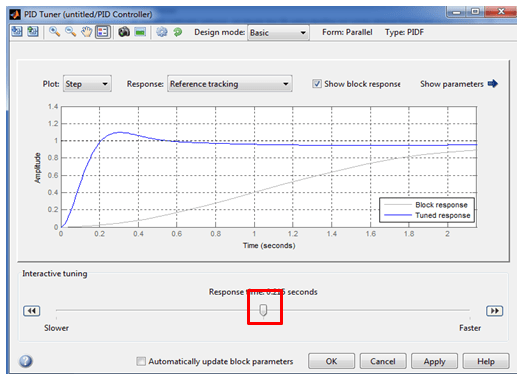Figure 7: Simulink Auto Tuning Window

Once you’ve tick the automatically update block parameters and then click OK the model will be updated automatically. The controller gain will be changed.

On the above window, we can only tune the controller but we cannot see the phase and gain margins. To view these margins you can drag a Gain and Phase margin Plot from a Simulink Control Design Library under Linear Analysis Plots. Below is the model with a Bode Plot.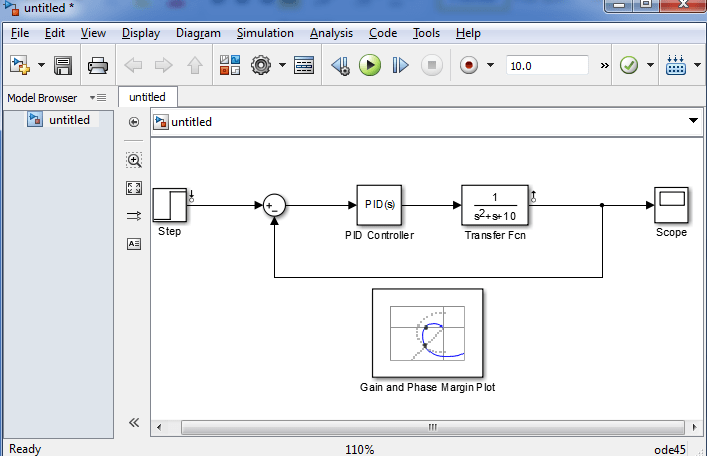Figure 8: Gain and Phase Margin

To know on how to setup the bode plot or the gain and phase margin plot in Simulink read this step by step tutorials.

Actually, Simulink control design could be in the top position of the tools that can be used in control system design because of its simplicity to use. However, Simulink is not free when you a Matlab license. This means that you are going to spend extra money for it. That’s why this is not as popular than Matlab.

### 3. Spice Based Simulation Software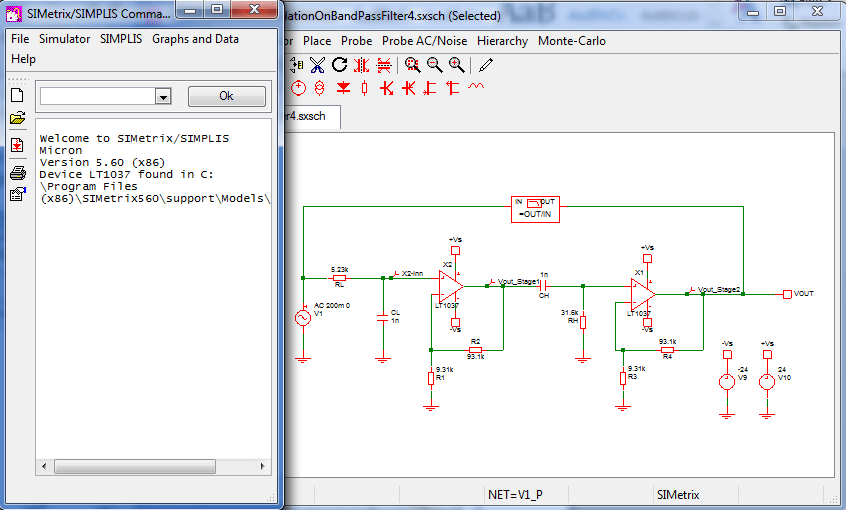Figure 9: Simetrix Window

Among the list of top tools that can be used in control system design I prefer Spice based simulator for electronic circuits. In my case since I am an SMPS engineer, I prefer using simetrix because of its speed advantage with simulation involving analog devices. With Simetrix or any spiced based simulator you don’t need to derive the transfer function anymore. However you need to still model the elements of the controller you want. Sometimes the model of the controller you need is already available in the net and this is a big help. This is also a trial and error approach until you arrived with the right gain and phase margins. Below is an example Bode Plot using simetrix.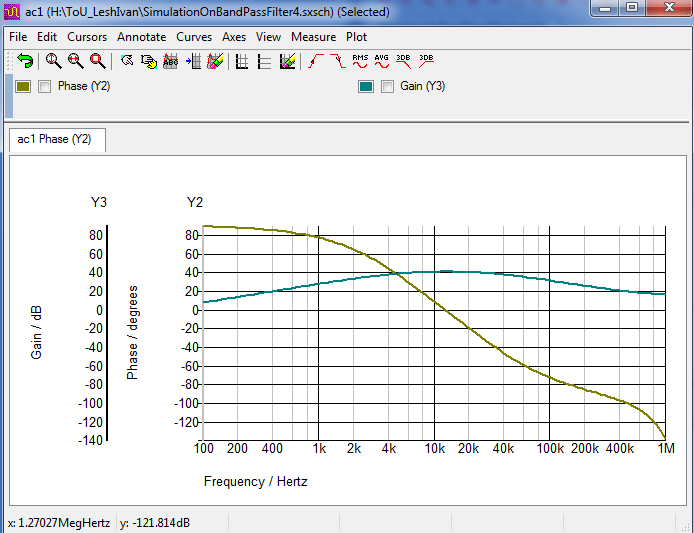Figure 10: Simetrix Gain and Phase Margin Plots

### 4. SimPowerSystems

For power electronics and other analog related circuits you can use SimPowerSystems. It is also under matlab or to be specific a matlab toolbox. Matlab is composed of many toolboxes that have specific function. And this particular toolbox is assigned for power electronics and electrical power systems. SimPowerSystems will also run under Simulink environment wherein you can drag and drop blocks and formed a circuit. In here you can draw a circuit just like how you draw it in spice based simulation software. Here is the simulation I made for Buck converter.Figure 11: Buck COnverter SimpowerSystems Simulation

The model consist of a reference, controller, plant, cyclic load and voltage sensors and scope.

This is how the plant is being modeled.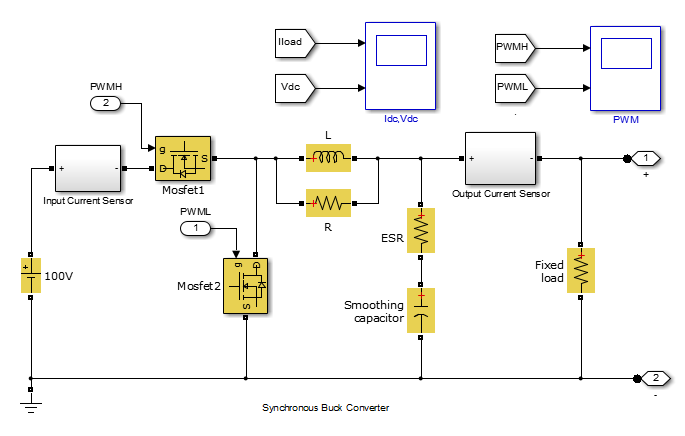Figure 12: Plant Model in simpowerSystems

As you can see, there are physical components like mosfets, inductor, capacitor and resistors. There is no need to derive the transfer function of the plant.

The controller is modeled like thisFigure 13: Controller Model in SimpowerSystems

This is a PI controller. This is a manually tuned controller unlike those with Simulink Control Design that you can make use of the auto tune feature. You can still use that auto tune as long as you can linearize the plant.

The PWM pulse source is modeled like this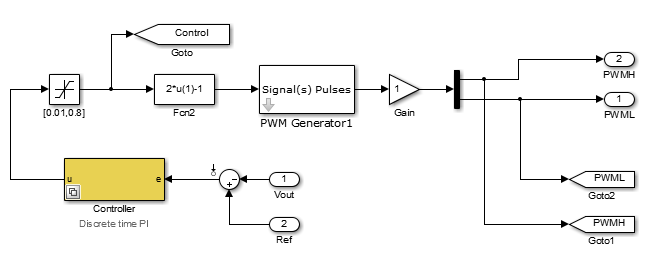Figure 14: PWM generator Mode in SimpowerSystems

Below is scope waveform when I run the circuit.Figure 15: Waveform Output in Simpowersystems

### 5. State Flow

An emerging tool nowadays for control system design is state flow. Most of the time this is used to design sophisticated control systems. This is of course more complicated than the previous methods. State flow is also a matlab toolbox. It can design sytems containing control, supervisory, and mode logic. It is an extension of Simulink design environment to develop state charts and flow diagrams. It can also provide fault management and scheduling. In below model, the block in yellow is state flow algorithm.Figure 16: State Flow Simulation

This is the content of the yellow blockFigure 17: State Flow Design

The concept of state flow is also used in the embedded systems and they called it state machines.

The last on the list of the tools that can be used in control system design is by using a Mathcad template. This is a tedious design approach. If you are the one starting the job, it requires advance knowledge in control systems and everything related to it. Such as poles and zeros, system bandwidth, gain and phase margin derivations etc. It requires formula derivation. However, if the template is already proven working, you may only change some values on it and you can see what you’re looking for.

If you want to know how to use Mathcad, read this step by step beginners guide.

Below is the design template I made for a type 2 control. Since the template is working already I just vary the values and the computation is automatically done.